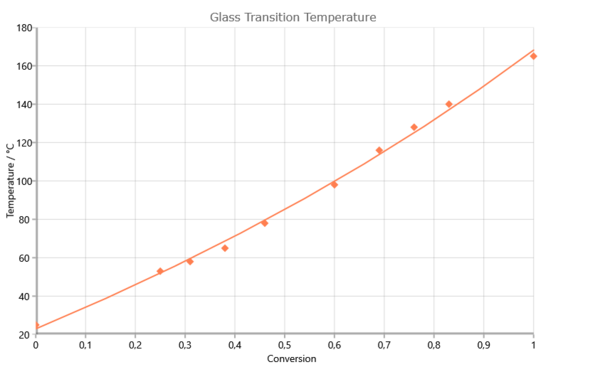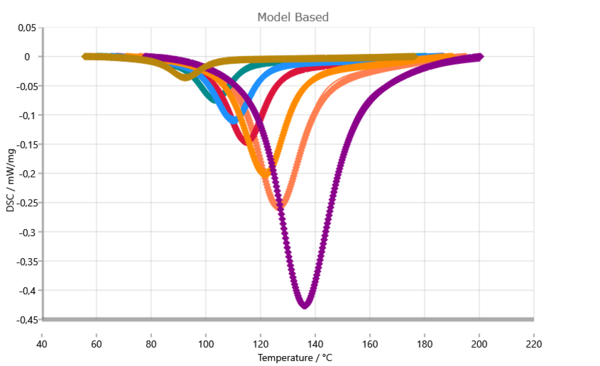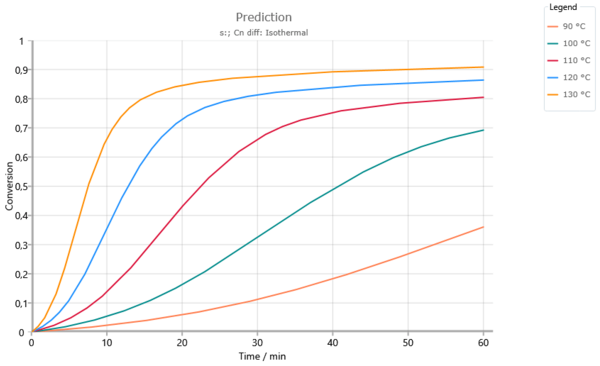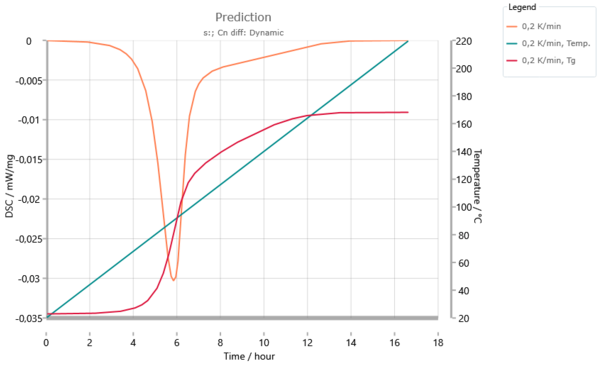Polimeri

# Epoxy Resin — Curing, Partial Diffusion Control

The speed of a chemical reaction, contributed by more than one reactant is controlled by two steps:

1. the speed of diffusion of the reactants together (characterized by Kdiff),

2. the speed of chemical reaction (characterized by Kchem).

The effective reaction speed is the geometric mean value of both speed constants:
1/Keff = 1/Kdiff + 1/Kchem (Rabinowitch equation).
It is obvious that Keff equals Kchem, if Kdiff >> Kchem is true.

Therefore, for the most part, the effect of diffusion control is not taken into account. If the reaction temperature is close to or smaller than the Temperatura di transizione vetrosaLa transizione vetrosa è una delle più importanti proprietà chimico-fisiche dei materiali amorfi e semi-cristallini, come, ad esempio, vetri, metalli (amorfi), polimeri, ingredienti farmaceutici e alimentari e definisce l’intervallo di temperatura in cui le proprietà meccaniche die materiali variano da duro e fragile a più morbido, malleabile o gommoso.glass transition temperature, then a strong increase of the viscosity is observed: the material under investigation vitrifies. Through the restricted mobility of reactants, the curing process is diffusion-controlled and Kchem >> Kdiff is true.

All calculations, modelling, fit and predictions for this application are made in NETZSCH Kinetics Neo software.

Dependence of Temperatura di transizione vetrosaLa transizione vetrosa è una delle più importanti proprietà chimico-fisiche dei materiali amorfi e semi-cristallini, come, ad esempio, vetri, metalli (amorfi), polimeri, ingredienti farmaceutici e alimentari e definisce l’intervallo di temperatura in cui le proprietà meccaniche die materiali variano da duro e fragile a più morbido, malleabile o gommoso.glass transition temperature on degree of reaction for the system 2,2¥,6,6¥-tetrabrom-bisphenol-A-diglycidylether (RUETAPOX VE 3579) + 5% Zn(OCN)2 [Flammersheim, Opfermann: Thermochim. Acta 337(1999)141]

The temperature dependence of Kchem is computed by the Arrhenius equation. Because Kdiff is inversely proportional to viscosity, its dependence on temperature is used. If (a) the basis of analysis are DSC measurements, then the glass transition temperature and its dependence on degree of reaction is used as the controlling value of viscosity. According to a special proposal, given by Wise [C.W.Wise, W.D.Cook, A.A.Goodwin: Polymer 38 (1997) 3251], the speed of diffusion is calculated by means of a modified Williams-Landel-Ferry (WLF) equation

For temperatures T lower than Tg the WLF equation is transformed to a Arrhenius equation under both conditions that both the transfer and the 1st derivation are continuous. The current activation energy for T<Tg is:

Otherwise, if (b) the basis of analysis are viscosity measurements, then the calculated viscosity is used as the controlling value. Now the viscosity is calculated by means of an Arrhenius equation with different activation energies for the uncured and cured material.

Comparision between measured (symbols) and calculated (solid lines) DSC curves.

Taking into account the diffusion control in the kinetic analysis, a nearly perfect fit is achieved. This high fit quality is the basic condition for predictions with a high level of confidence.

Isothermal predictions for temperatures below glass transition temperature Tg = 165°C. The increase of the degree of reaction kinks where the glass transition temperature reaches the reaction temperature (see following picture). Without the use of diffusion control above 120°C full conversion would already be achieved after 60 min.

This information becomes understandable by means of the following picture, a simulation for the heating rate 0.2 K/min: the glass transition temperature reaches the reaction temperature after 6 hours. From here up to a reaction time of 12 hours, so much reacts that the increase of the glass transition temperature equals the increase of the reaction temperature. In this range the reaction is diffusion-controlled.

Dynamic prediction for a heating rate of 0.2 K/min. The glass transition temperature reaches the reaction temperature after 6 hours. The DSC signal breaks down except for a constant value. Above 12 hours the glass transition temperature Tg increases less than the reaction temperature. The system stops the "vitrifying" condition.Fig.1. Dependence of glass transition temperature on degree of conversion.Fig.2. Measured data(symbols) and Kinetic model (solid lines) for curing of epoxy resin with diffusions control.Fig.3. prediction of degree of conversion for isothermal condition, 60 min.Fig.4. Predicted DSC data (orange) and glass transition temperature (red) for heating from 20°C to 220°C with 0.2K/min